# 烘焙百分比算法，用数学与逻辑找到开启烘焙大门的这把“小钥匙”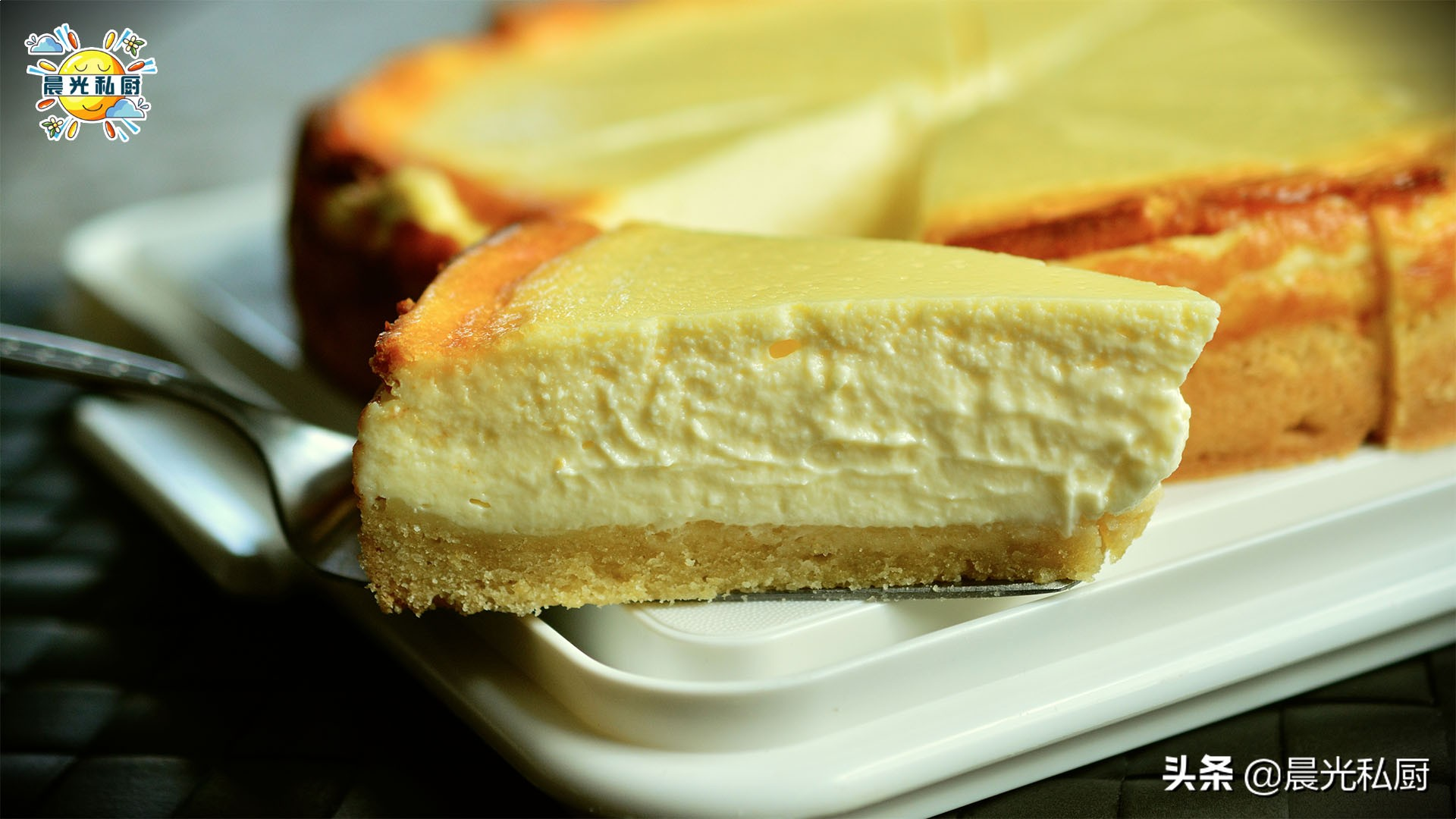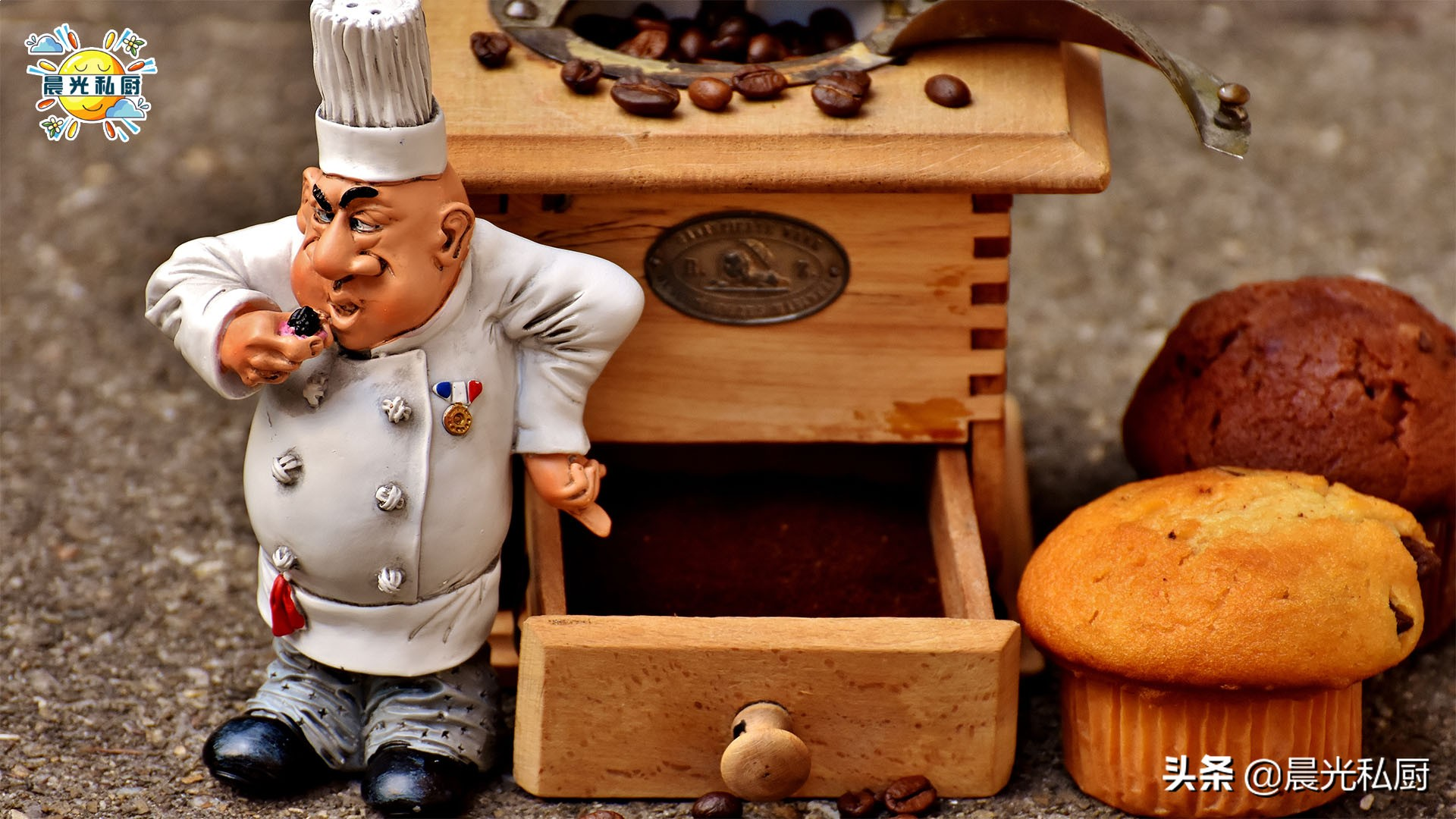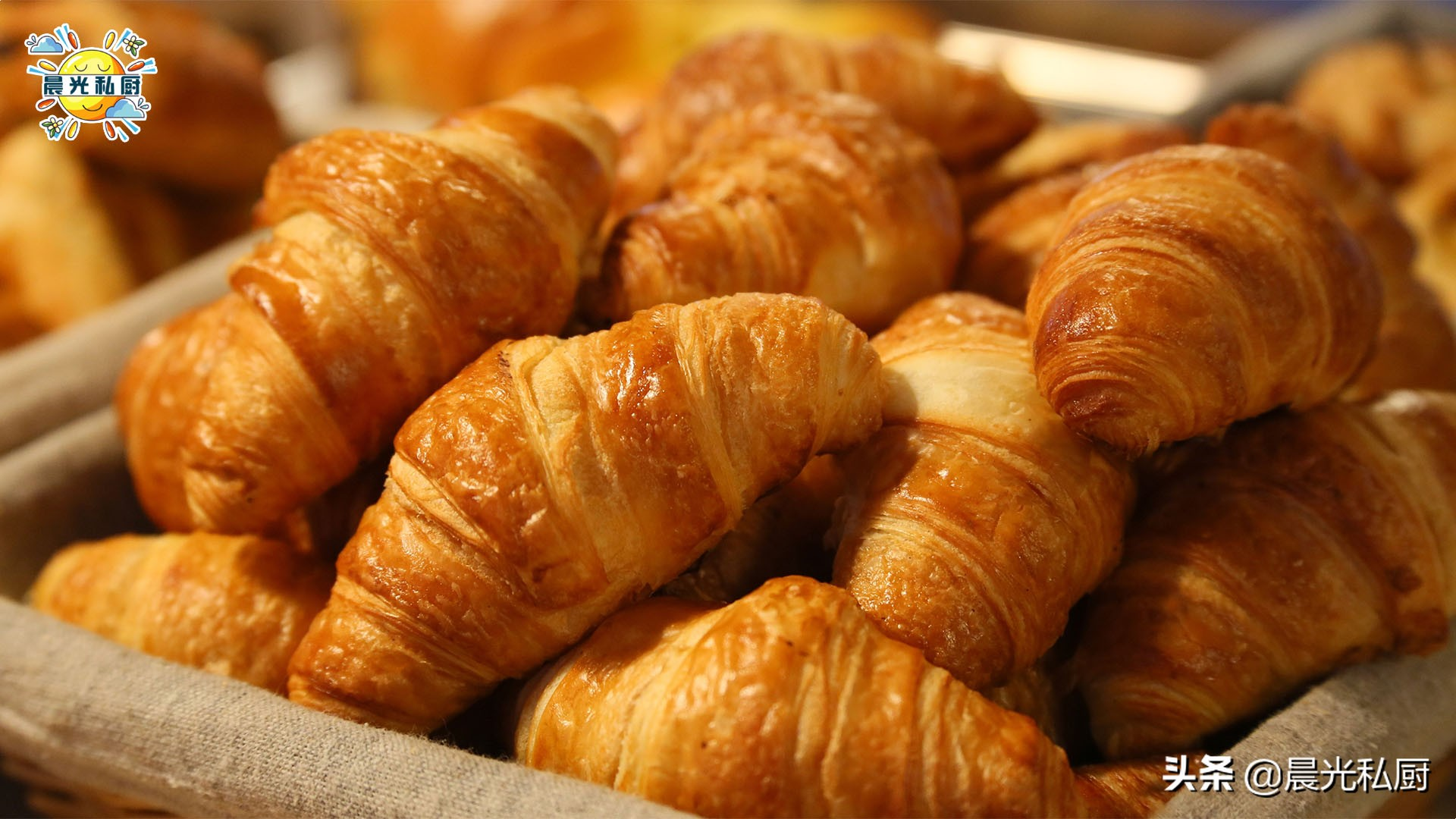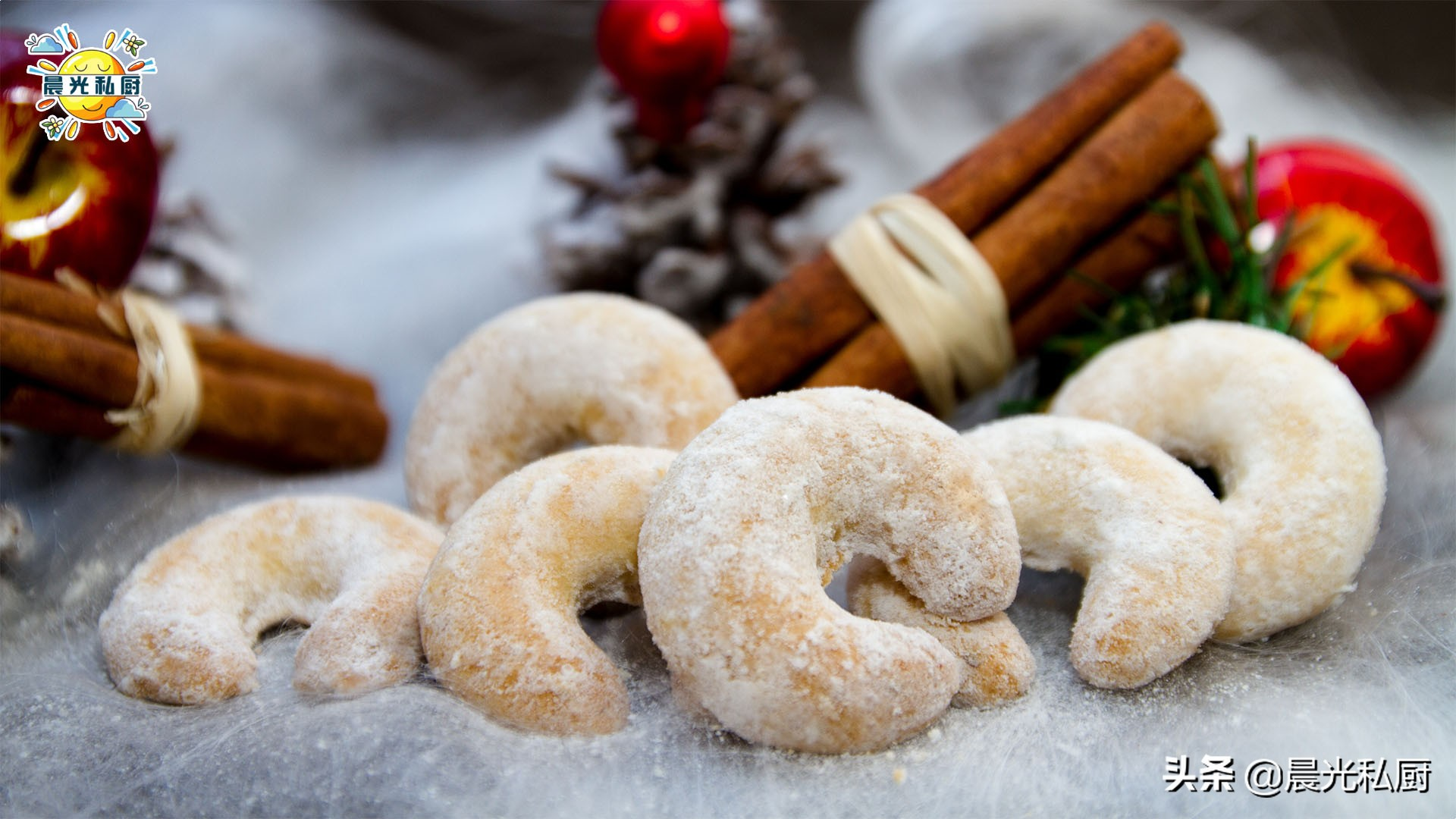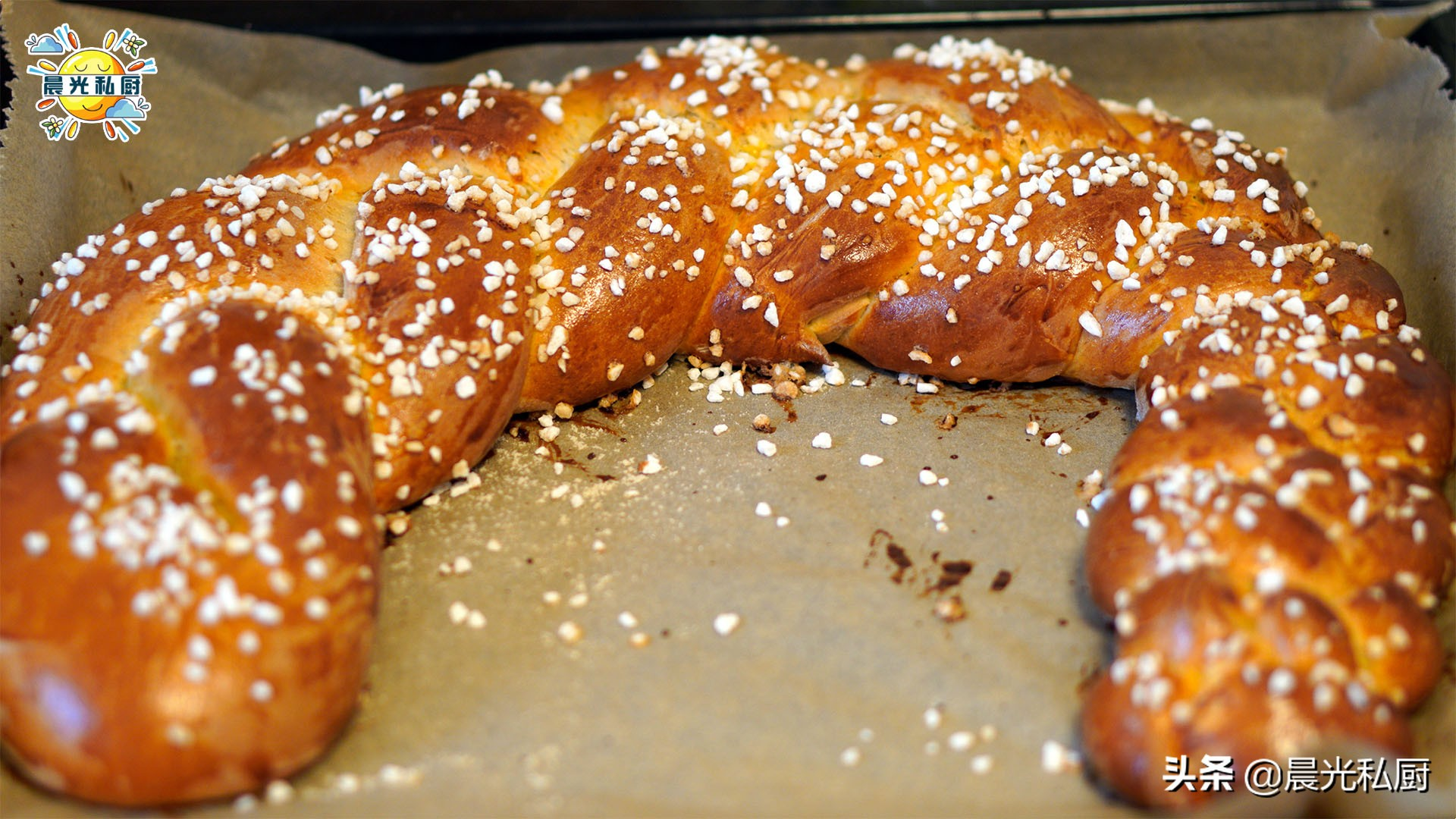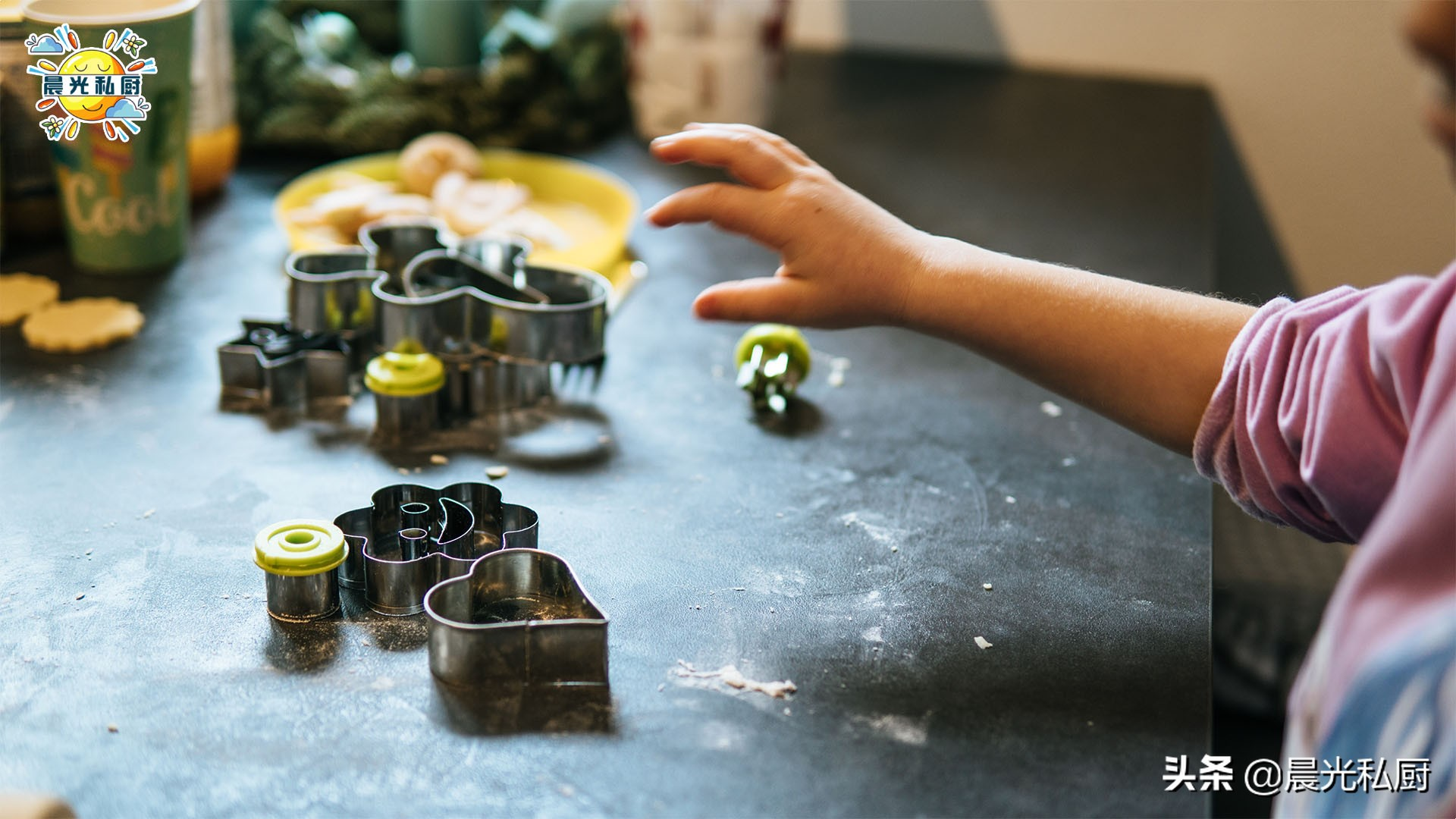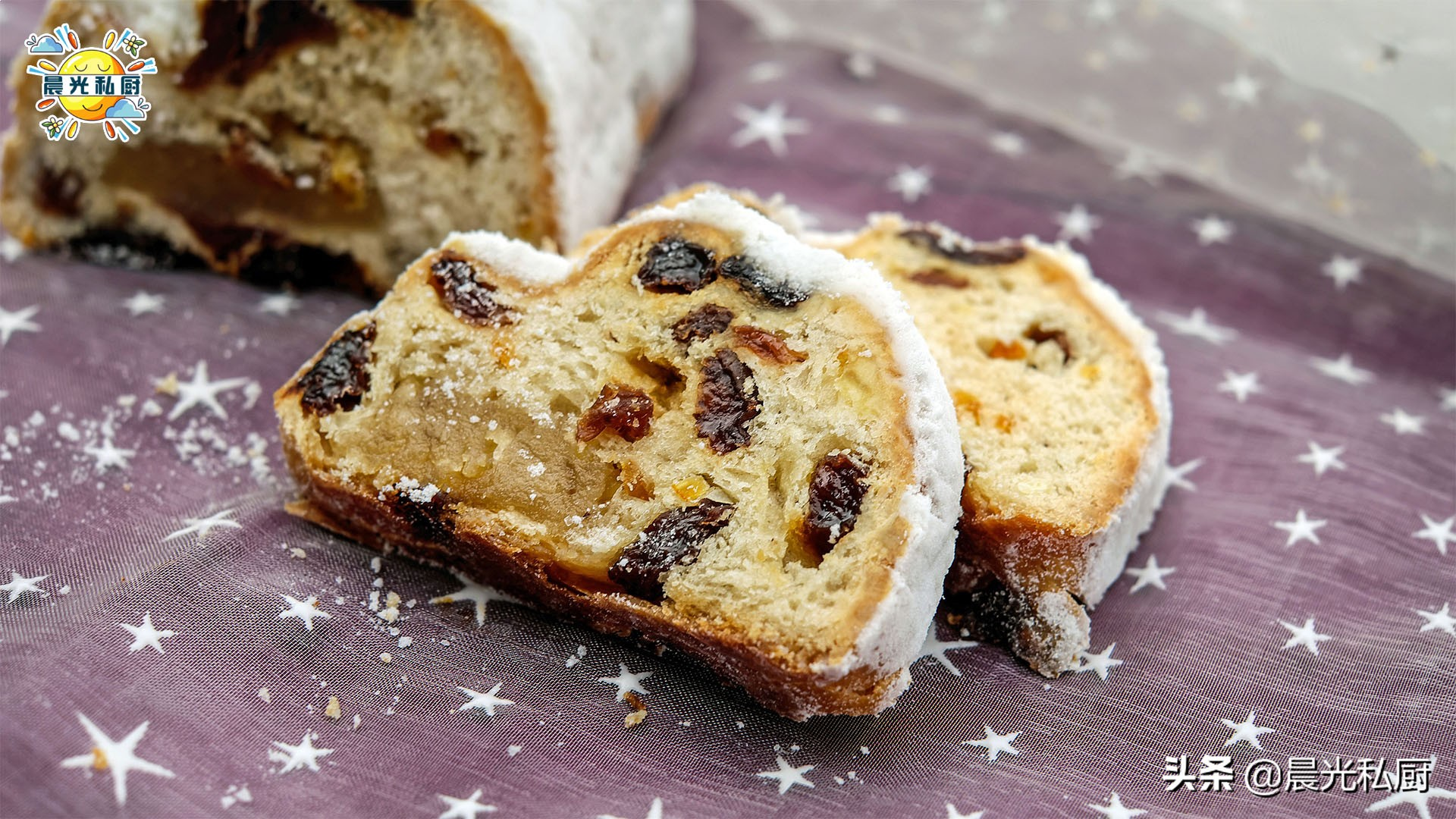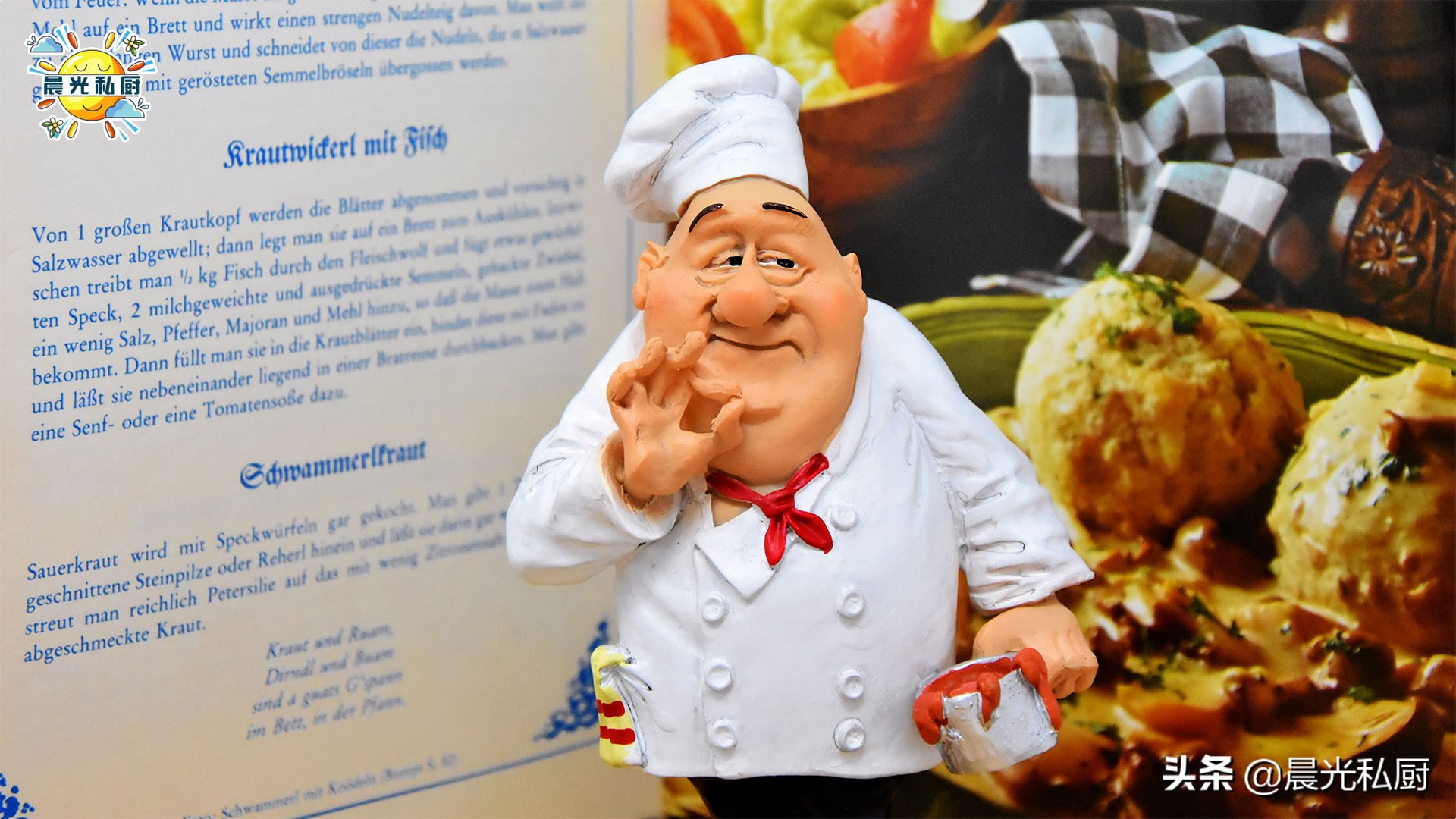• 高筋面粉 100%
• 细砂糖 8%
• 食盐 1%
• 速发干酵母 1%
• 无盐黄油 8%
• 全脂纯牛奶 72%
• 动物性淡奶油 25%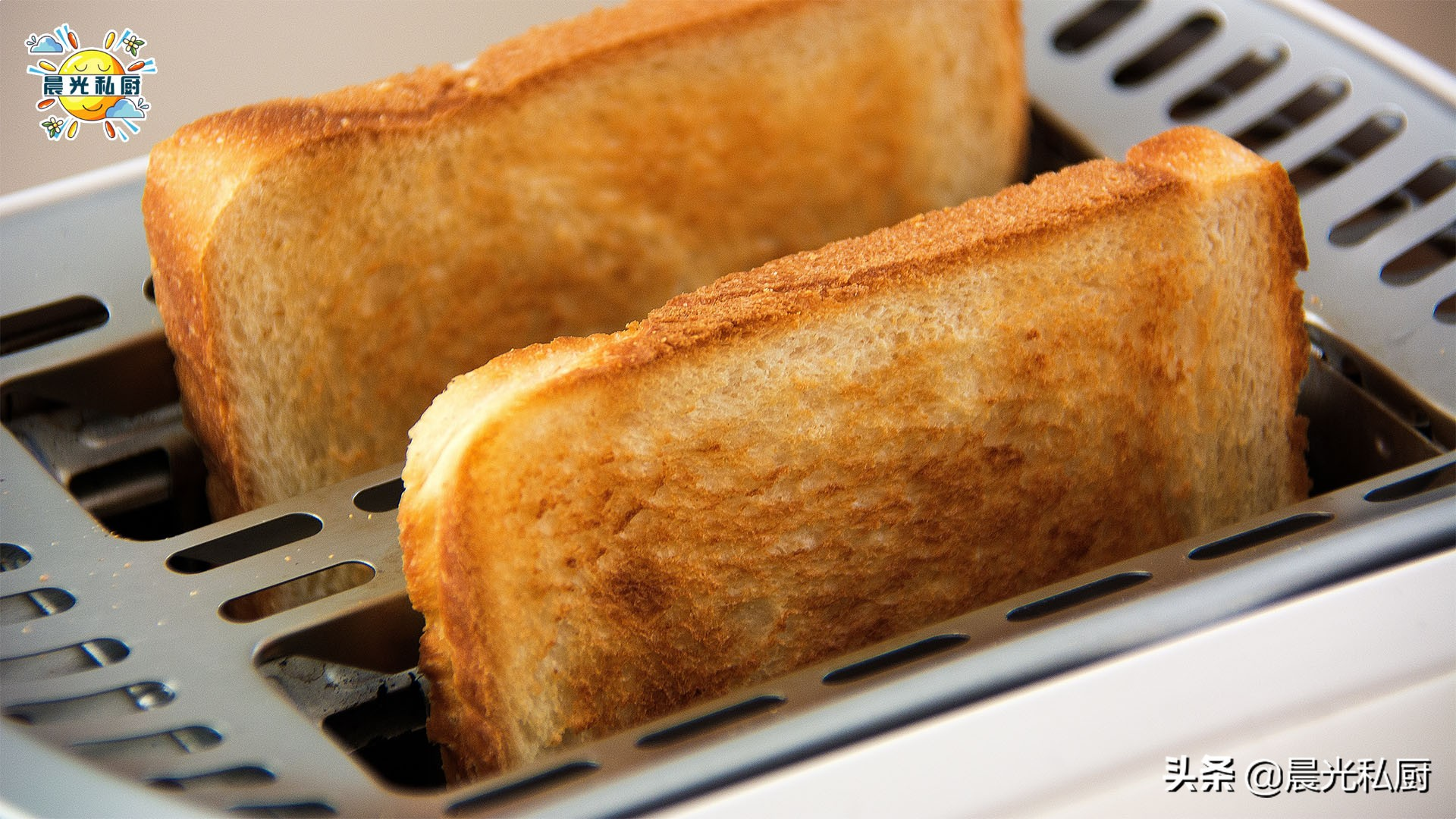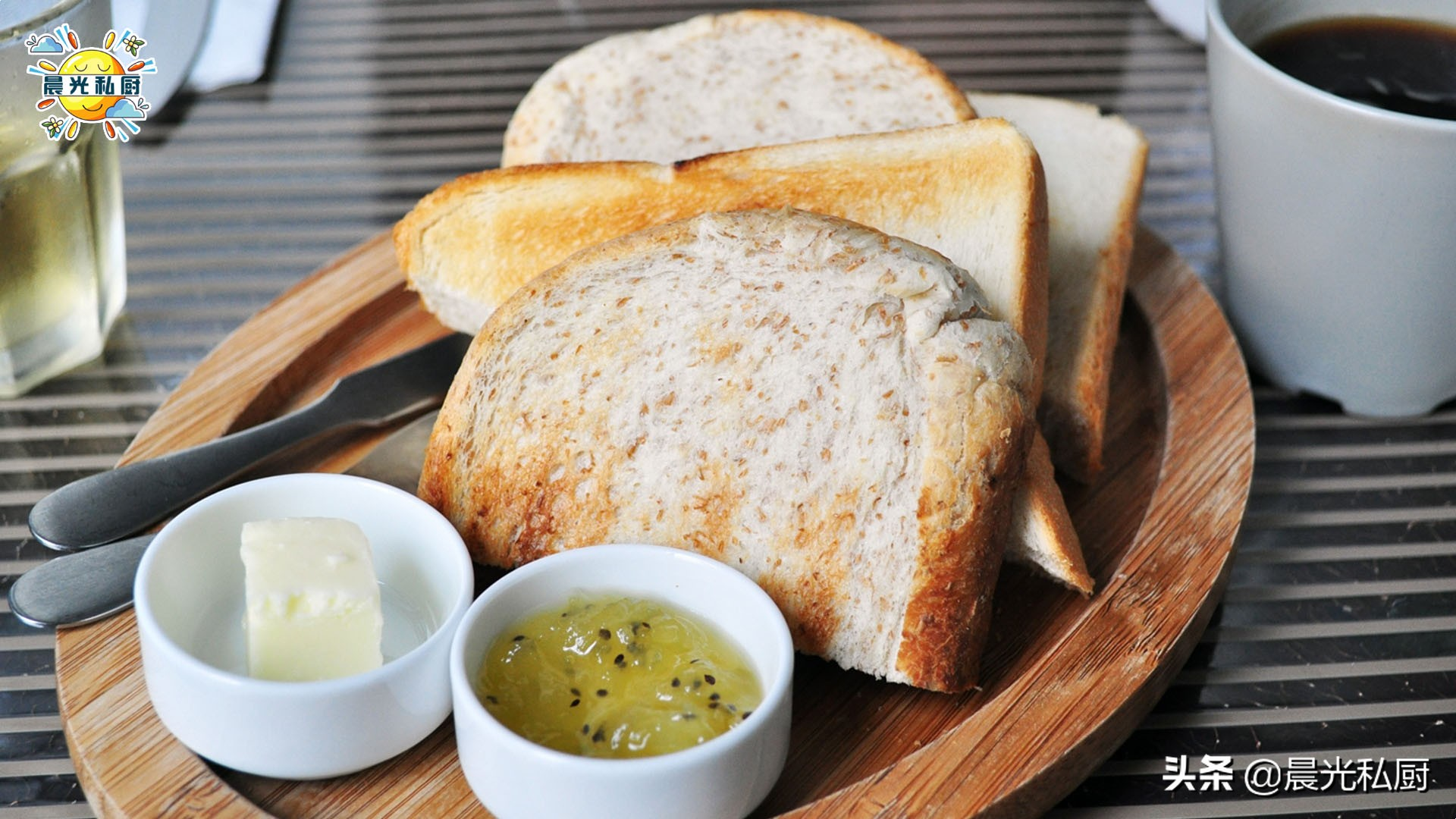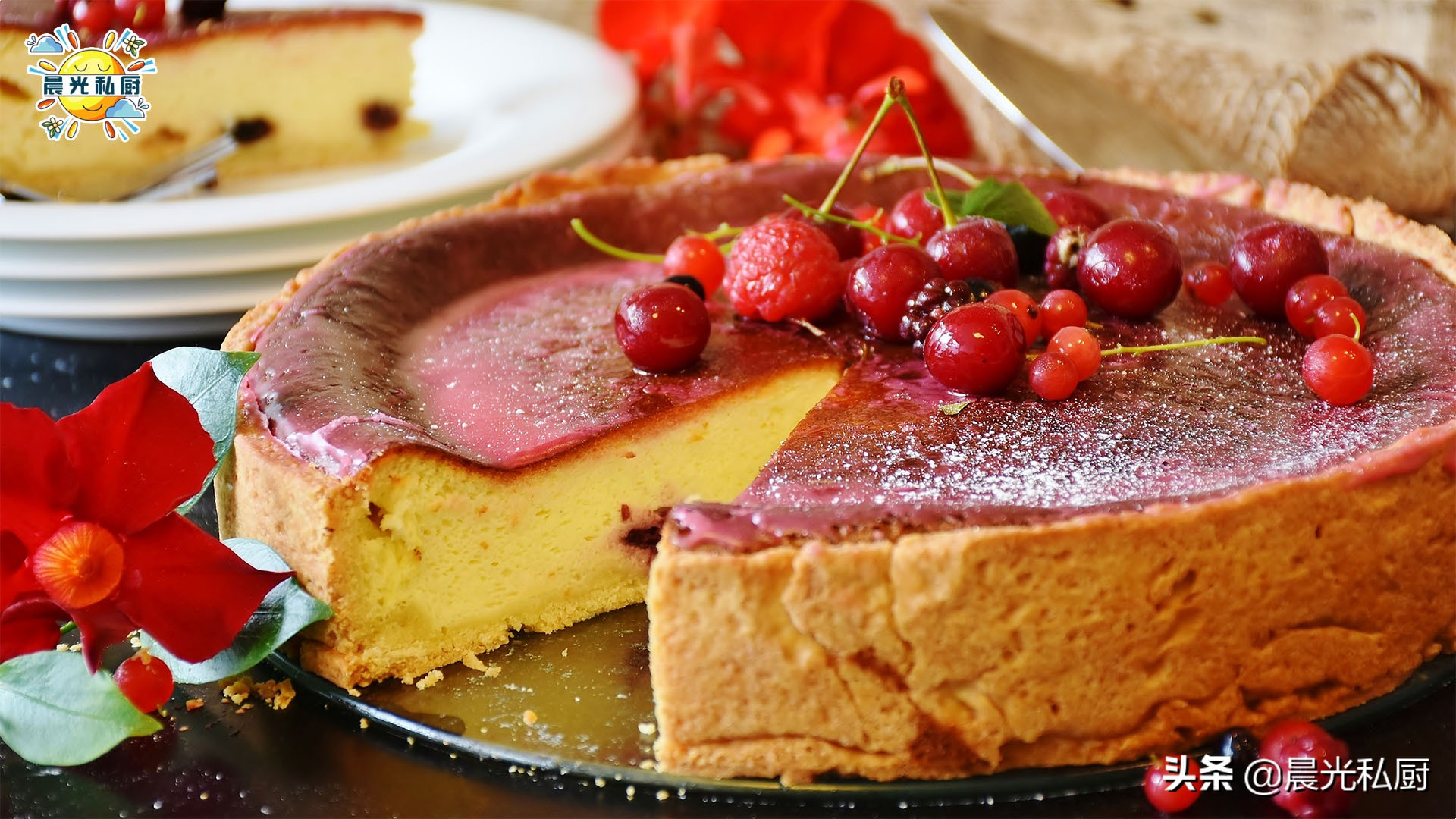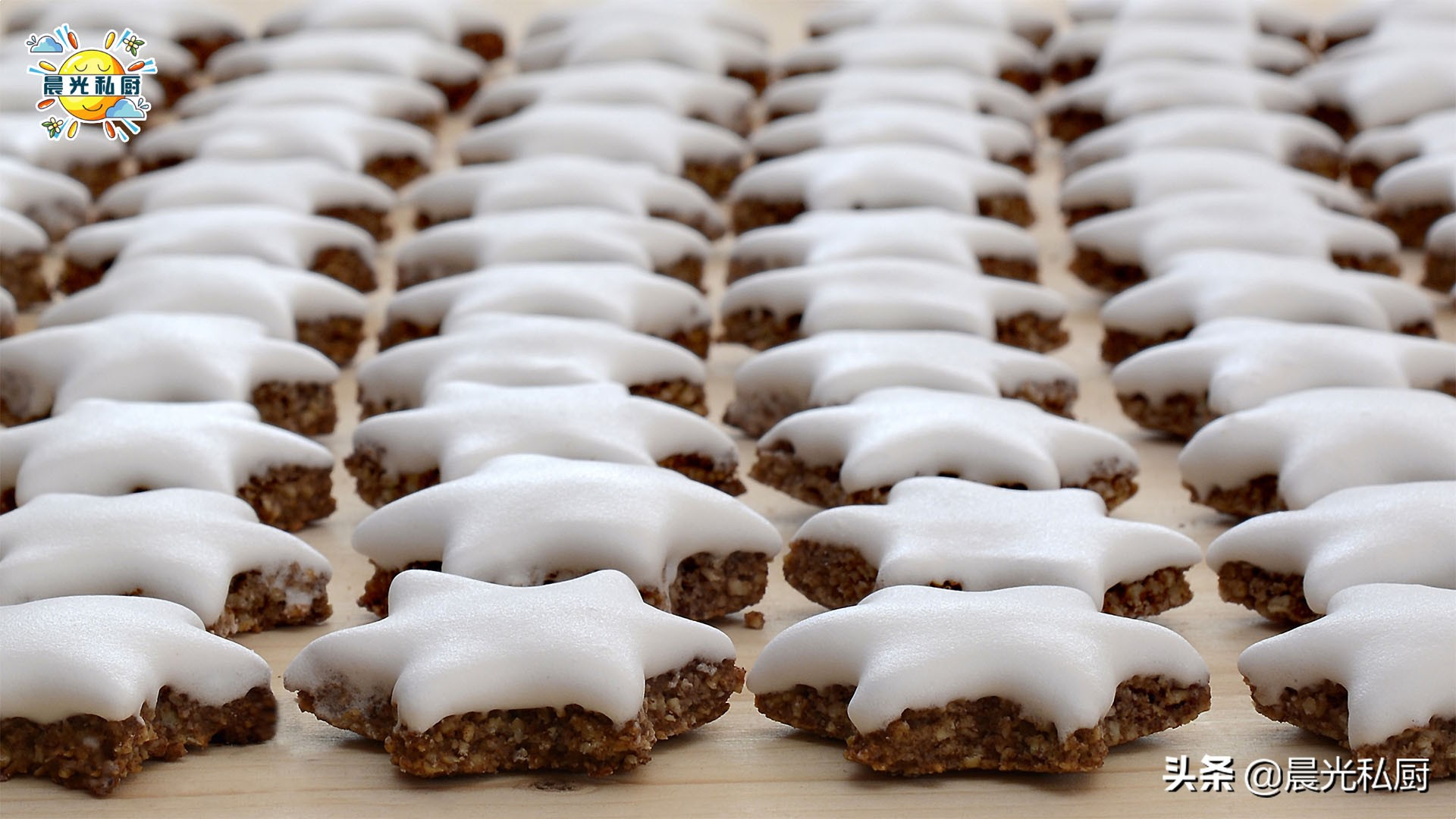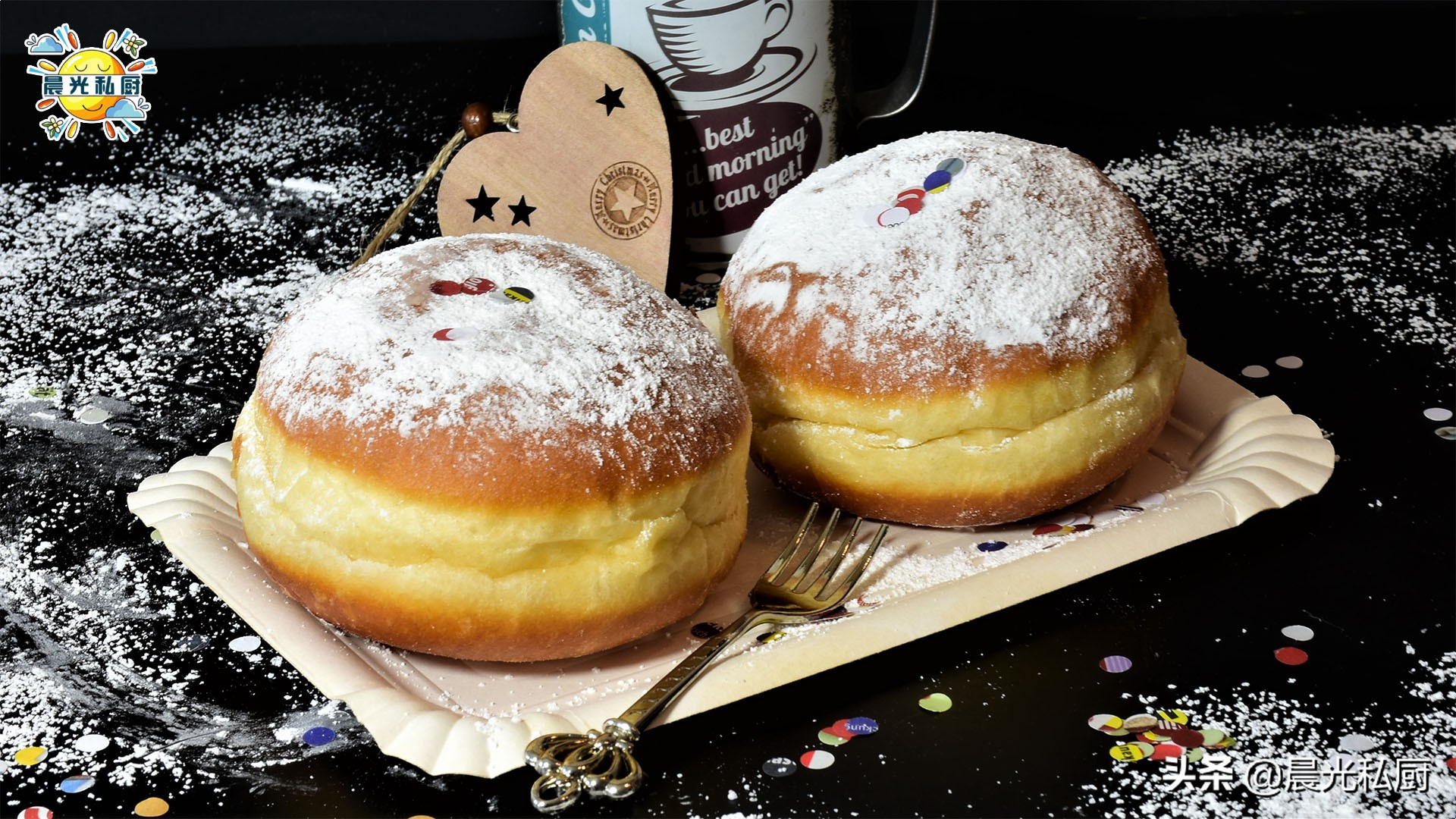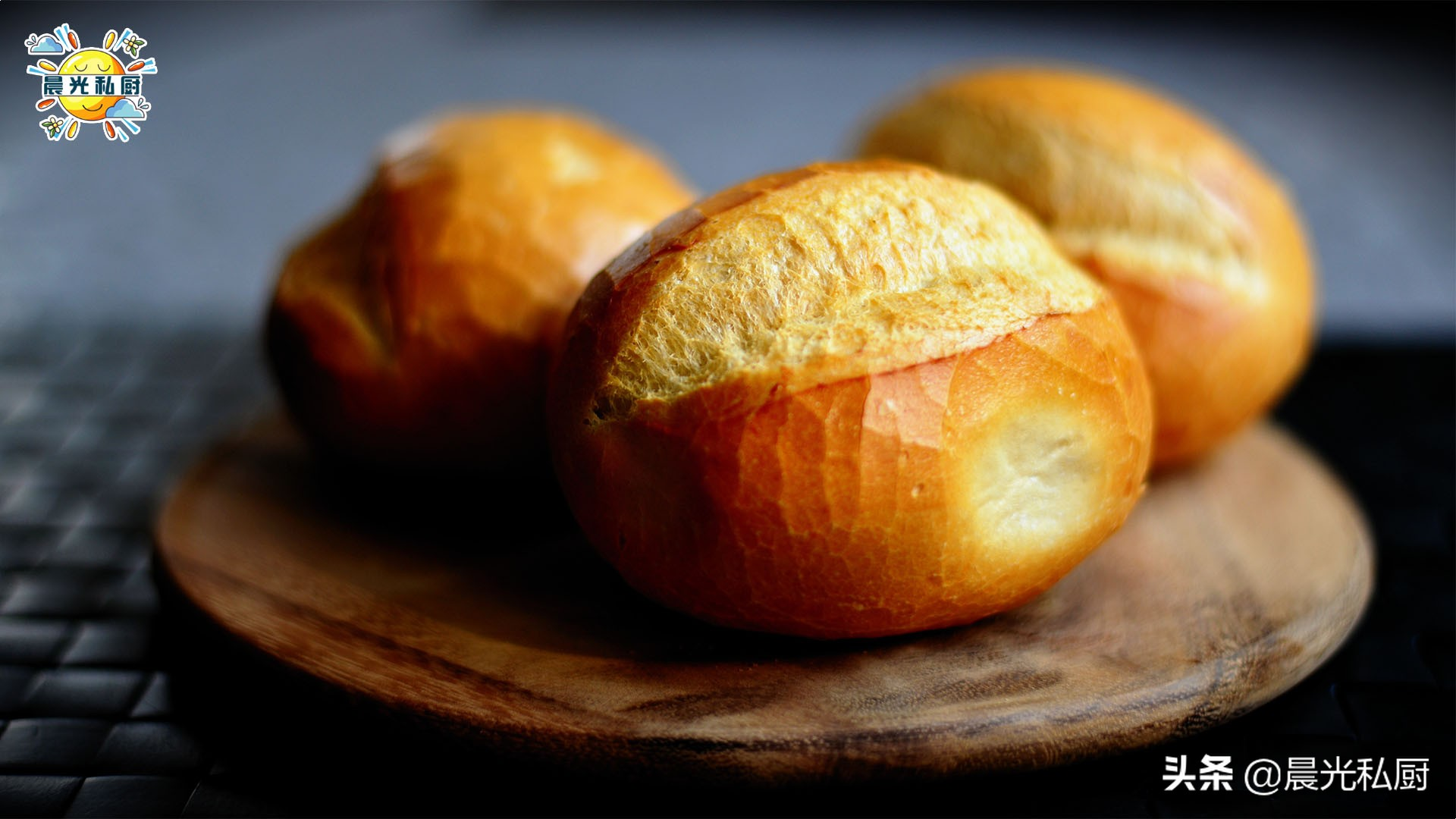• 高筋面粉 100%×2.51=251克
• 细砂糖 8%×2.51=20克（20.08）
• 食盐 1%×2.51=2.5克（2.51）
• 速发干酵母 1%×2.51=2.5克（2.51）
• 无盐黄油 8%×2.51=20克（20.08）
• 全脂纯牛奶 72%×2.51=181克（180.72）
• 动物性淡奶油 25%×2.51=63克（62.75）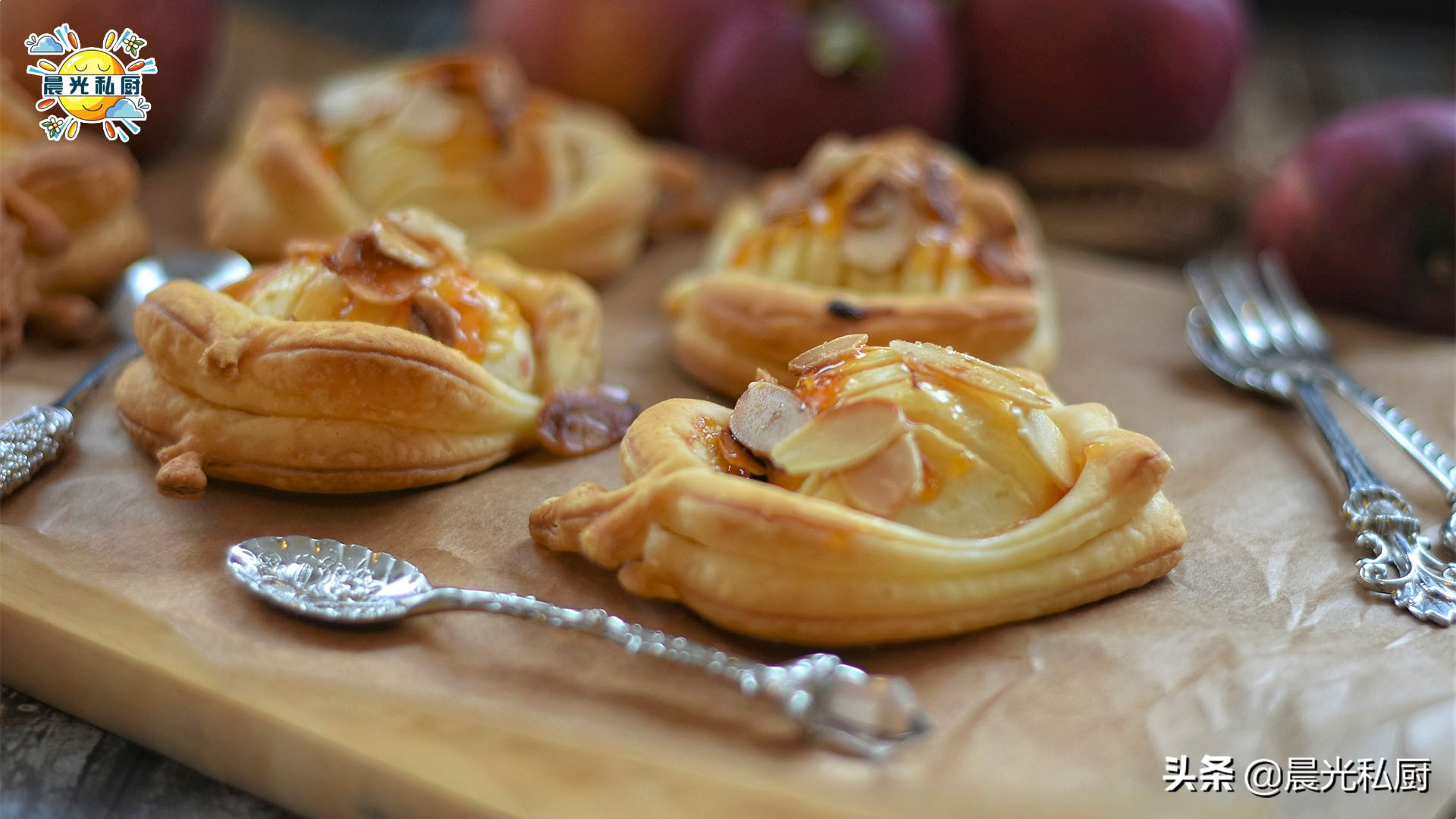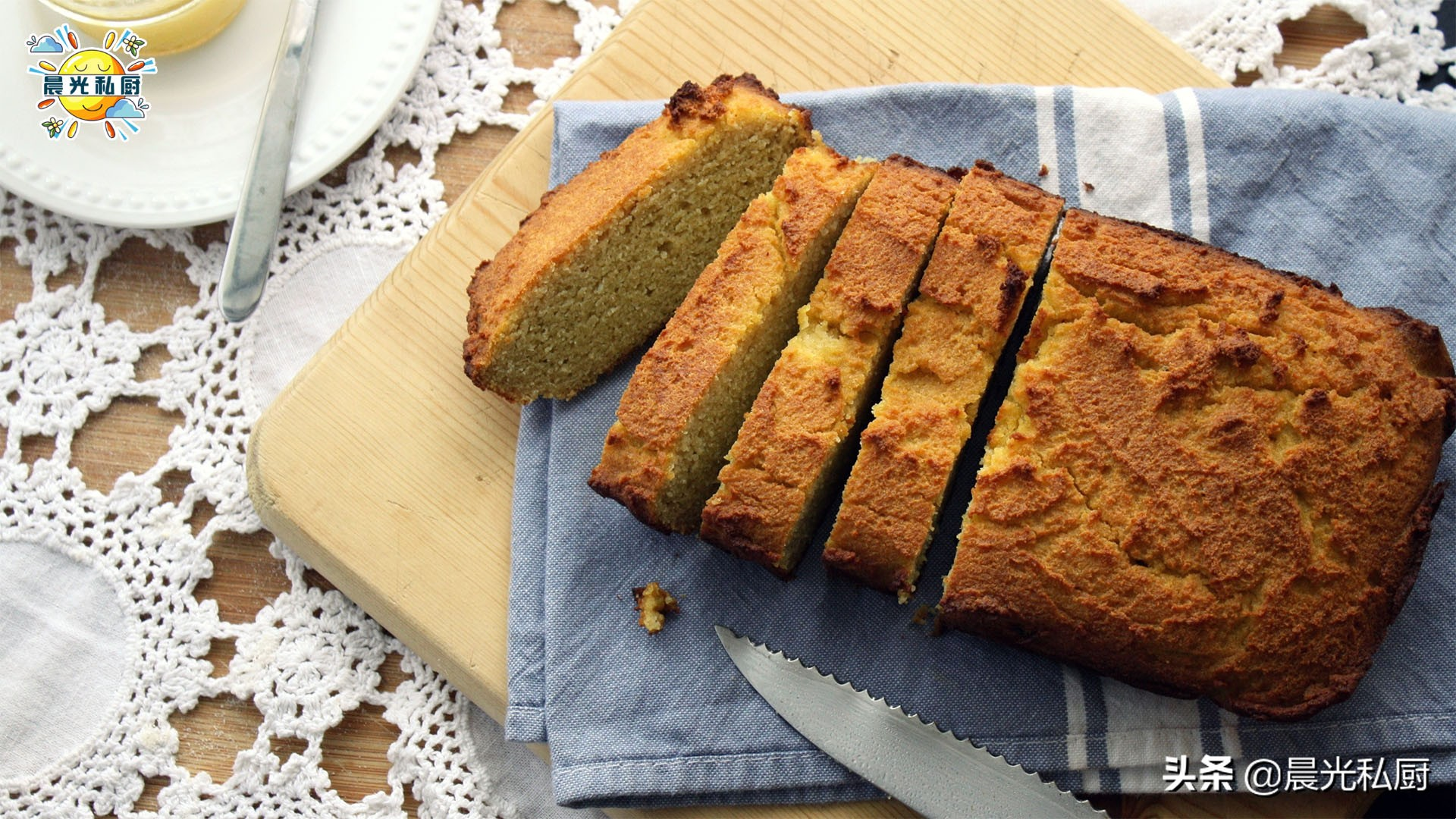• 高筋面粉 100%×2.98=298克
• 细砂糖 8%×2.98=24克（23.84）
• 食盐 1%×2.98=3克（2.98）
• 速发干酵母 1%×2.98=3克（2.98）
• 无盐黄油 8%×2.98=24克（23.84）
• 全脂纯牛奶 72%×2.98=215克（214.56）
• 动物性淡奶油 25%×2.98=75克（74.5）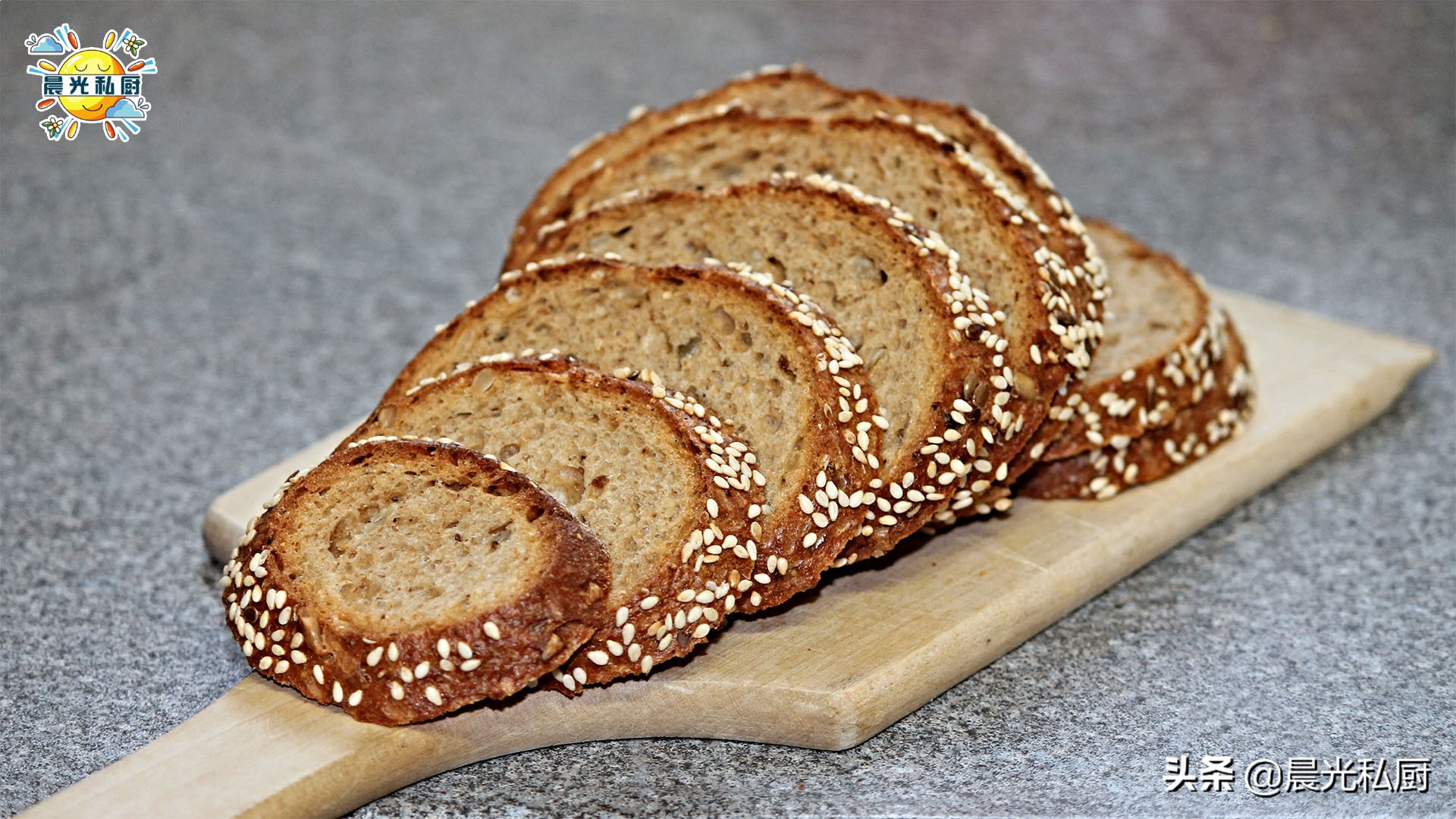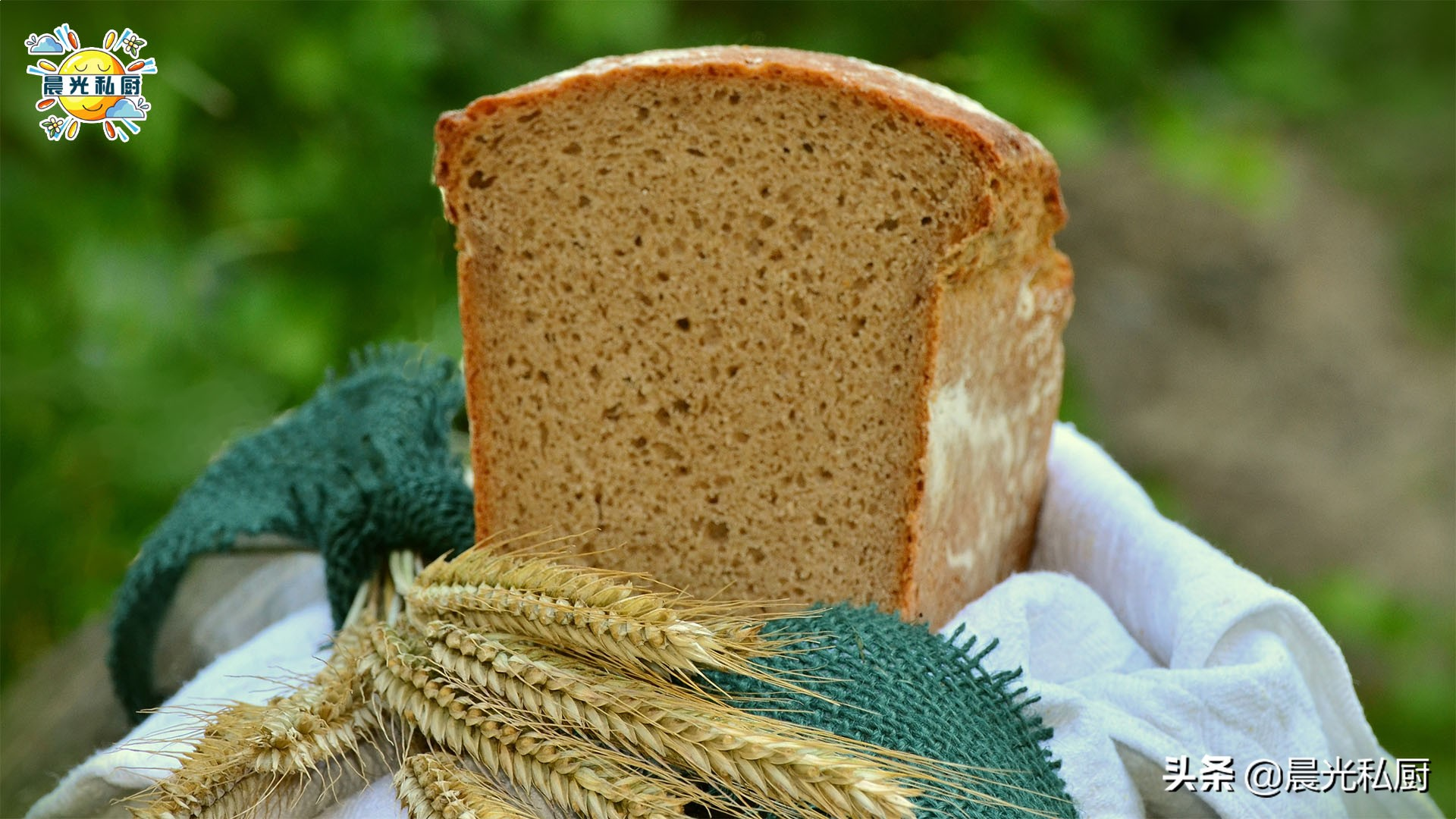# 晨光碎碎念